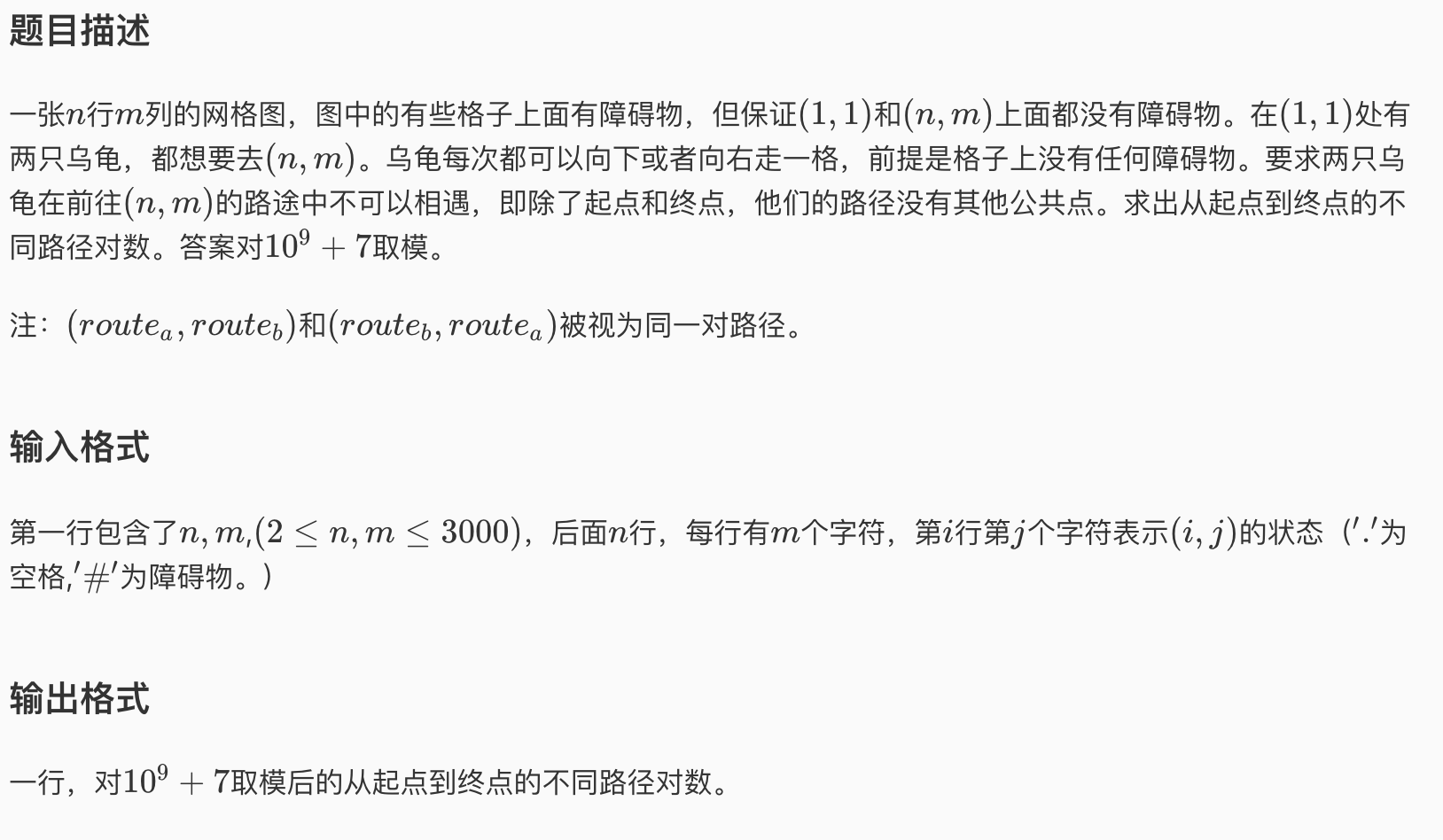#社论

LGV定理告诉我们，在二维空间下，有起点集 $S$，以及终点集 $T$，从 $S$ 到 $T$ 的每一个点走到对应点且不相交路径的方案数为下列矩阵的行列式：

$$\begin{pmatrix} f(S_1,T_1) & f(S_1,T_2) & f(S_1,T_3) & \cdots & f(S_1,T_n) \\ f(S_2,T_1) & f(S_2,T_2) & f(S_2,T_3) & \cdots & f(S_2,T_n) \\ \vdots & \vdots & \vdots & \ddots & \vdots \\ f(S_n,T_1) & f(S_n,T_2) & f(S_n,T_3) & \cdots & f(S_n,T_n) \end{pmatrix}$$

posted @ 2019-07-03 19:35  KingSann  阅读(...)  评论(...编辑  收藏